# Investment Analysis and Portfolio Management Lecture 2 a

• Slides: 8Investment Analysis and Portfolio Management Lecture 2 a Gareth MylesReturn on a short sale l l l The calculation of the return for a short sales raises some questions Considering this issue gives an insight into the meaning of “return” Assume an investor sells short 100 stock at a price of £ 1 each A year later the short sale is reversed when the stock are trading at £ 0. 50 What is the return?Return on a short sale l l l It is clear the investment has been successful The investor received £ 100 at the time of the short sale The borrowed stock were replaced for £ 50 The investor has gained £ 50 So the return must be positive?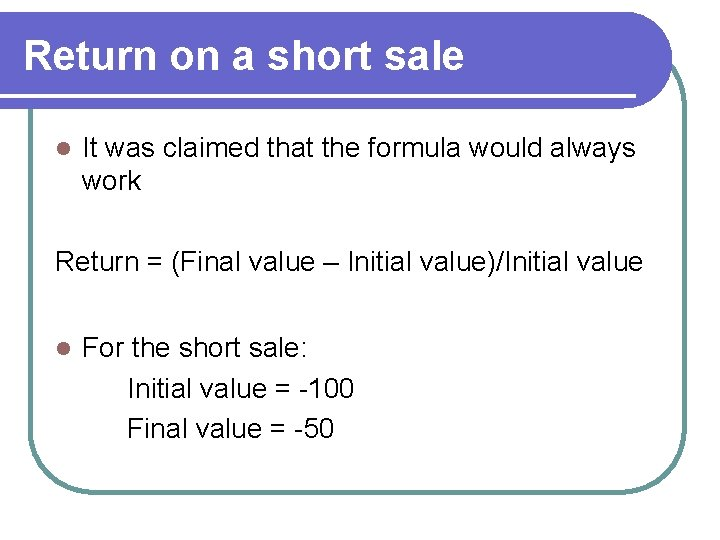Return on a short sale l It was claimed that the formula would always work Return = (Final value – Initial value)/Initial value l For the short sale: Initial value = -100 Final value = -50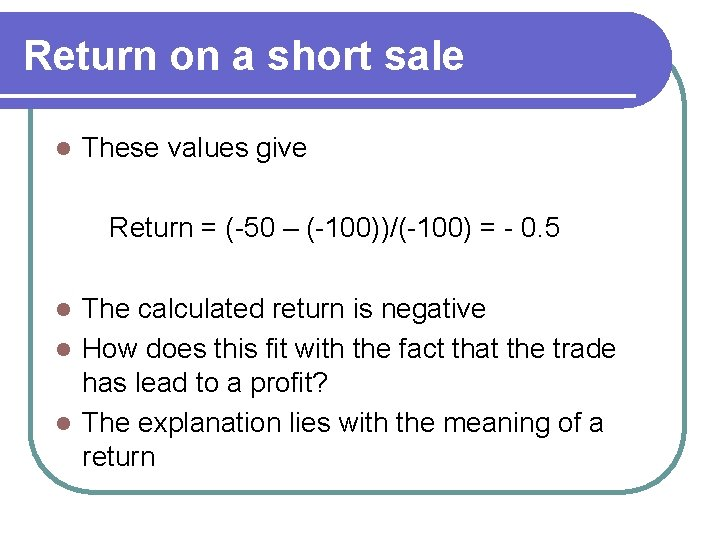Return on a short sale l These values give Return = (-50 – (-100))/(-100) = - 0. 5 The calculated return is negative l How does this fit with the fact that the trade has lead to a profit? l The explanation lies with the meaning of a return l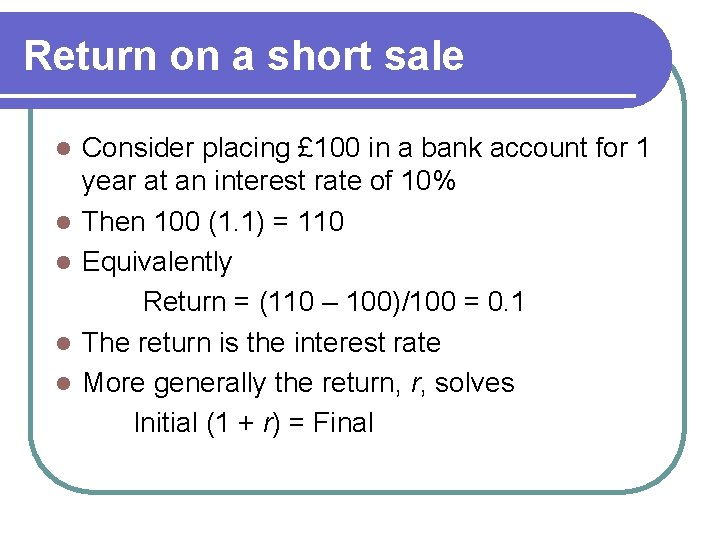Return on a short sale l l l Consider placing £ 100 in a bank account for 1 year at an interest rate of 10% Then 100 (1. 1) = 110 Equivalently Return = (110 – 100)/100 = 0. 1 The return is the interest rate More generally the return, r, solves Initial (1 + r) = Final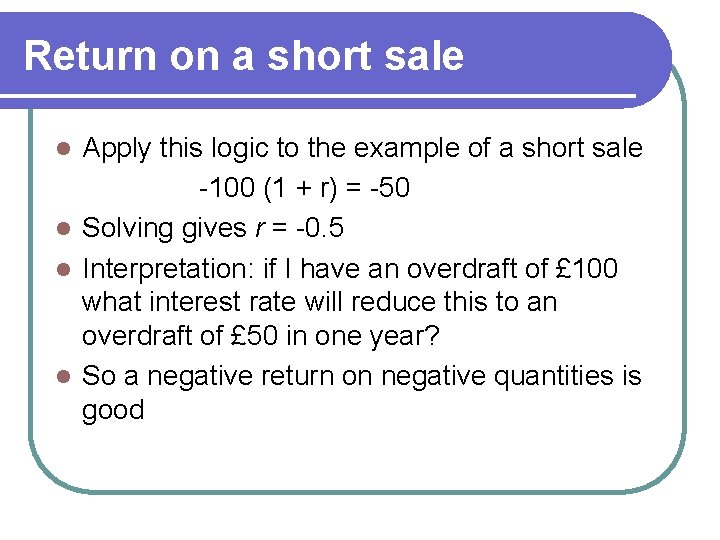Return on a short sale Apply this logic to the example of a short sale -100 (1 + r) = -50 l Solving gives r = -0. 5 l Interpretation: if I have an overdraft of £ 100 what interest rate will reduce this to an overdraft of £ 50 in one year? l So a negative return on negative quantities is good l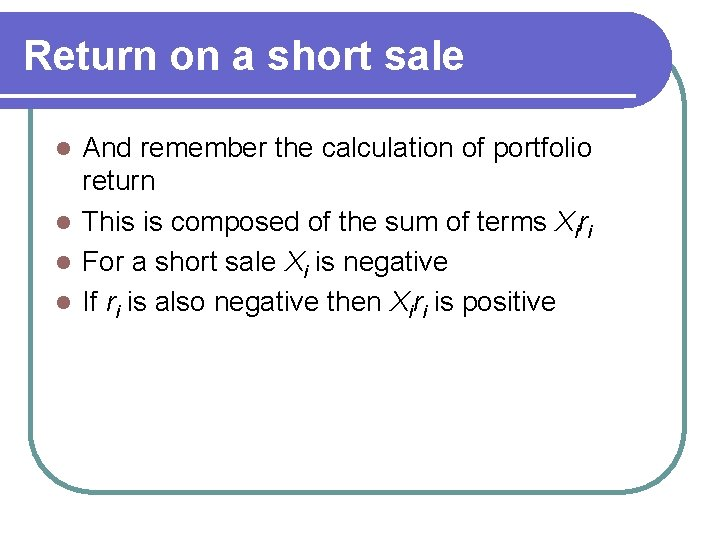Return on a short sale And remember the calculation of portfolio return l This is composed of the sum of terms Xiri l For a short sale Xi is negative l If ri is also negative then Xiri is positive l